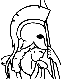#Nonlinear Programming Table of Contents:

1. Unconstrained Optimization: Basic Methods

1. Optimality Conditions
1. Variational Ideas
2. Main Optimality Conditions
1. Descent Directions and Stepsize Rules
2. Convergence Results
3. Gradient Methods -- Rate of Convergence
1. The Local Analysis Approach
2. The Role of the Condition Number
3. Convergence Rate Results
4. Newton's Method and Variations
1. Modified Cholesky Factorization
2. Trust Region Methods
3. Variants of Newton's Method
4. Least Squares and the Gauss-Newton Method
5. Notes and Sources

1. Conjugate Direction Methods
2. Convergence Rate of Conjugate Gradient Method
2. Quasi-Newton Methods
3. Nonderivative Methods
1. Coordinate Descent
2. Direct Search Methods
4. Incremental Methods
3. Incremental Gauss-Newton Methods
4. Incremental Newton Methods
5. Distributed Asynchronous Algorithms
1. Totally and Partially Asynchronous Algorithms
2. Totally Asynchronous Convergence
4. Convergence Rate of Asynchronous Algorithms
6. Discrete-Time Optimal Control
2. Newton's Method for Optimal Control
7. Solving Nonlinear Programming Problems - Some Practical Guidelines
8. Notes and Sources

3. Optimization Over a Convex Set

1. Constrained Optimization Problems
1. Necessary and Sufficient Conditions for Optimality
2. Existence of Optimal Solutions
2. Feasible Directions - Conditional Gradient Method
1. Descent Directions and Stepsize Rules
1. Feasible Directions and Stepsize Rules Based on Projection
2. Convergence Analysis
4. Two-Metric Projection Methods
5. Manifold Suboptimization Methods
6. Proximal Algorithms
1. Rate of Convergence
2. Variants of the Proximal Algorithm
7. Block Coordinate Descent Methods
1. Variants of Coordinate Descent
8. Network Optimization Algorithms
9. Notes and Sources

4. Lagrange Multiplier Theory

1. Necessary Conditions for Equality Constraints
1. The Penalty Approach
2. The Elimination Approach
3. The Lagrangian Function
2. Sufficient Conditions and Sensitivity Analysis
1. The Augmented Lagrangian Approach
2. The Feasible Direction Approach
3. Sensitivity
3. Inequality Constraints
1. Karush-Kuhn-Tucker Optimality Conditions
2. Sufficient Conditions and Sensitivity
3. Fritz John Optimality Conditions
4. Constraint Qualifications and Pseudonormality
5. Abstract Set Constraints and the Tangent Cone
6. Abstract Set Constraints, Equality, and Inequality Constraints
4. Linear Constraints and Duality
1. Convex Cost Functions and Linear Constraints
2. Duality Theory: A Simple Form for Linear Constraints
5. Notes and Sources

5. Lagrange Multiplier Algorithms

1. Barrier and Interior Point Methods
1. Path Following Methods for Linear Programming
2. Primal-Dual Methods for Linear Programming
2. Penalty and Augmented Lagrangian Methods
1. The Quadratic Penalty Function Method
2. Multiplier Methods -- Main Ideas
3. Convergence Analysis of Multiplier Methods
4. Duality and Second Order Multiplier Methods
5. Nonquadratic Augmented Lagrangians - The Exponential Method of Multipliers
3. Exact Penalties - Sequential Quadratic Programming
1. Nondifferentiable Exact Penalty Functions
3. Differentiable Exact Penalty Functions
4. Lagrangian Methods
1. First-Order Lagrangian Methods
2. Newton-Like Methods for Equality Constraints
3. Global Convergence
4. A Comparison of Various Methods
5. Notes and Sources

6. Duality and Convex Programming

1. Duality and Dual Problems
1. Geometric Multipliers
2. The Weak Duality Theorem
3. Primal and Dual Optimal Solutions
4. Treatment of Equality Constraints
5. Separable Problems and Their Geometry
2. Convex Cost - Linear Constraints
3. Convex Cost - Convex Constraints
4. Conjugate Functions and Fenchel Duality
1. Conic Programming
2. Monotropic Programming
3. Network Optimization
4. Games and the Minimax Theorem
5. The Primal Function and Sensitivity Analysis
5. Discrete Optimization and Duality
1. Examples of Discrete Optimization Problems
2. Branch-and-Bound
3. Lagrangian Relaxation
6. Notes and Sources

7. Dual Methods

2. Dual Dual Ascent Methods for Differentiable Dual Problems
1. Coordinate Ascent for Quadratic Programming
2. Separable Problems and Primal Strict Convexity
3. Partitioning and Dual Strict Concavity
3. Proximal and Augmented Lagrangian Methods
1. The Method of Multipliers as a Dual Proximal Algorithm
2. Entropy Minimization and Exponential Method of Multipliers
3. Incremental Augmented Lagrangian Methods
4. Alternating Direction Methods of Multipliers
1. ADMM Applied to Separable Problems
2. Connections Between Augmented Lagrangian-Related Methods
2. Approximate and Incremental Subgradient Methods
3. Cutting Plane Methods
4. Ascent and Approximate Ascent Methods
6. Decomposition Methods
1. Lagrangian Relaxation of the Coupling Constraints
2. Decomposition by Right-Hand Side Allocation
7. Notes and Sources

8. Appendix A: Mathematical Background

9. Appendix B: Convex Analysis

10. Appendix C: Line Search Methods

11. Appendix D: Implementation of Newton's Method

12. References

13. Index